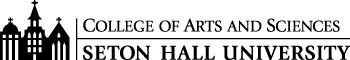# B.S. Financial Mathematics/M.S. Financial Analytics Curriculum

#### Freshman Year

CSAS 1114 Introduction to Program Design I 3
MATH 1501 Calculus I for Mathematics & Physical Sciences I 4
MATH 1511 Calculus for the Mathematical and Physical Sciences II 4
MATH 1611 Introductory Discrete Mathematics 3
PHIL 1204 Symbolic Logic* (recommended) 3
ECON 1402 Principles of Economics 3
ECON 1403 Principles of Economics II 3

#### Sophomore Year

MATH 2511 Calculus for the Mathematical and Physical Sciences III 4
MATH 2711 Introduction to Probability and Statistics 4
MATH 2813 Linear Algebra 4
BACC 2103 Financial Accounting 3

#### Junior-Senior Year

MATH 3515 Analysis 4
MATH3711 Statistical Analysis I 3
MATH 6721 Financial Calculus I 3
MATH 7722 Adv. Topics in Financial Calculus 3
BFIN 4 of the following 5 courses:
BFIN 3211 Financial Strategy 4
BFIN 4227 Investment Analysis 3
BFIN 4250 Fixed Income Analysis 3
BFIN 4253 Advanced Corporate Finance 3
BFIN 4255 Financial Modeling 3

#### Junior-Senior Year Courses Taken Towards the M.S. in Financial Analytics

• Take the graduate course DAVA 7000 Data Visualization which will count towards FE550 Data Visualizations Applications in the M.S. in Financial Analytics.
• Take MATH 6611 Operations Research that counts towards FE646 Optimization Models and Methods in Finance.
• Take the graduate course MATH 6721 Financial Calculus I (required for B.S. in Financial Mathematics) that counts towards FE530 Introduction to Financial Engineering for the M.S. in Financial Analytics.
• Take the graduate course MATH 7722 Financial Calculus II (required for B.S. in Financial Mathematics) which counts towards FE543 Introduction to Stochastic Calculus for Finance for the M.S. in Financial Analytics as well as the B.S. in Financial Mathematics.

#### Last Year as Graduate in M.S. in Financial Analytics

Foundation Courses (a total of 12 credits)

1. FE511 Introduction to Bloomberg and Thomson Reuters (1 credits)
2. FE515 Introduction to R (1 credits)
3. FE520 Introduction to Python for Financial Applications (1 credits)
4. FE582 Foundations of Financial Data Science (2 credits) + FE513 Financial Lab: Database Design (1 credit)
5. FE541 Applied Statistics with Applications in Finance
6.  Choose one course from the following (3 credits total):
• FE530 Introduction to Financial Engineering (Credit from Seton Hall MATH 6721)
• FE535 Introduction to Financial Risk Management
• FE540 Probability theory for Financial Engineering
• FE620 Pricing and Hedging
• FE543 Introduction to Stochastic Calculus for Finance (Credit from Seton Hall MATH 7722)
• FIN510 Financial Statement Analysis
7. Data Visualization (3 credits). Choose one course from the following:
• FE550 Data Visualization Applications (Credit/Prep from Seton Hall DAVA 7000)
• FE555 2D Data Visualization Programming for Financial Applications
8. Time Series (3 credits). Choose one course from the following:
• FE542 Time Series with Applications to Finance
• MA641 Time Series Analysis
• FIN620 Financial Econometrics
• FE641 Multivariate Statistics and Advanced Time Series in Finance
9. Machine Learning (3 credits). Choose one course from the following:
• FE590 Statistical Learning in Finance
• BIA656 Statistical Learning and Analytics
• FE690 Machine Learning in Finance
10. Big Data (3 credits). Choose one course from the following:
• FE595 Financial Technology (FinTech)
• BIA678 Big Data Analytics
11. Optimization (3 credits). Choose one course from the following:
• FE630 Portfolio Theory and Applications
• FE646 Optimization Models and Methods in Finance (Credit/Prep from Seton Hall MATH 6611)
• MA629 Convex Analysis and Computation
12. FE800 Project in Financial Engineering (3 credits) - to be taken in the last semester

The MSFA program requires completion of 30 credits. Other courses may be substituted to those listed pending the Advisor's approval.